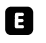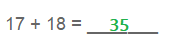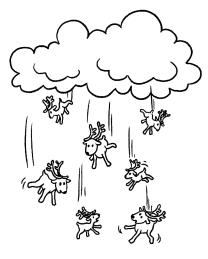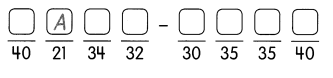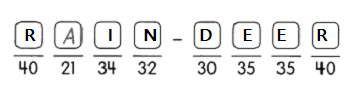Practice the problems of Math in Focus Grade 1 Workbook Answer Key Chapter 13 Practice 2 Addition with Regrouping to score better marks in the exam.

Fill in the missing numbers.

Example
23 + 9 = 2 tens 3 ones + 9 ones
= 2 tens 12 ones
= 32

Question 1.
37 + 3 = ____ tens ___ ones + ___ ones
= ____ tens ____ ones
= _____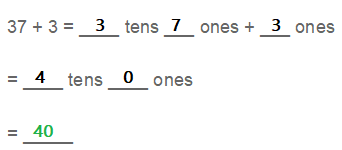Question 2.
25 + 8 = ____ tens ___ ones + ___ ones
= ____ tens ____ ones
= _____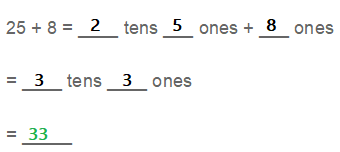Question 3.
26 + 6 = ____ tens ___ ones + ___ ones
= ____ tens ____ ones
= _____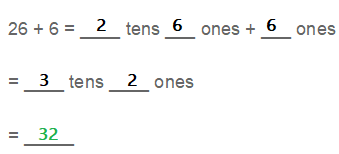Fill in the missing numbers.

Question 4.
5 + 29 = ____ ones + ___ tens + ___ ones
= ____ tens ____ ones
= _____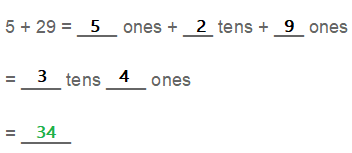Question 5.
19 + 21 = ____ ten + ___ ones + ___ tens ___ ones
= ____ tens ____ ones
= _____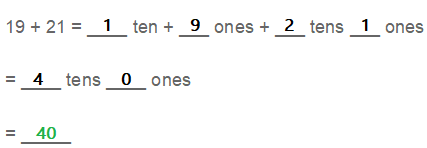Question 6.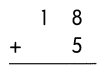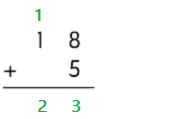Question 7.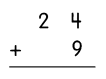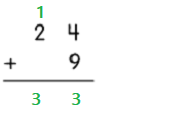Question 8.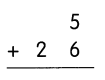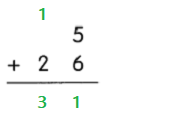Question 9.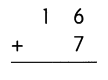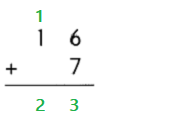Question 10.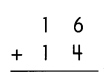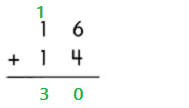Question 11.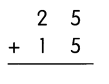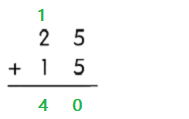Fill in the missing numbers.

Question 12.
18 + 7 = ____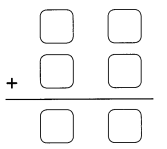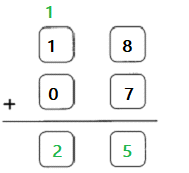Question 13.
21 + 9 = ____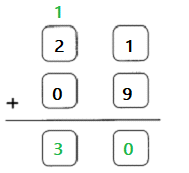Question 14.
6 + 15 = ____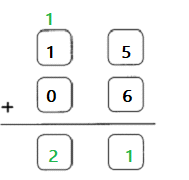Question 15.
8 + 32 = ___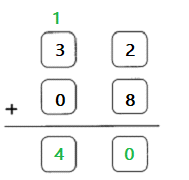Question 16.
17 + 16 = ___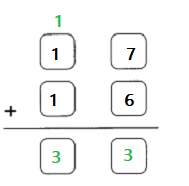Question 17.
13 + 19 = ___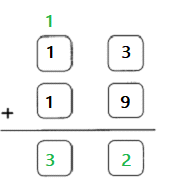Question 18.
14 + 7 = 21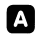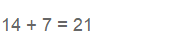Question 19.
26 + 8 = ____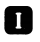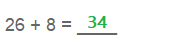Question 20.
29 + 6 = ___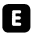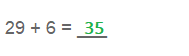Question 21.
23 + 9 = ___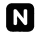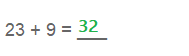Question 22.
33 + 7 = ___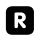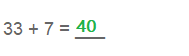Question 23.
18 + 22 = ___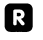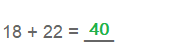Question 24.
6 + 24 = _______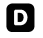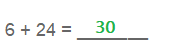Question 25.
17 + 18 = _______